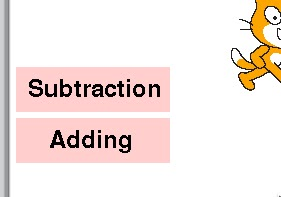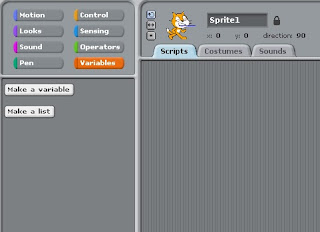## Posts

Showing posts with the label Scratch

### Which Programming Language Should I Learn First?By McDonald. T |  July 29th 2018 If you are thinking of learning how to code, the number of different types of programming languages can be a little daunting.  Putting it another way, there are languages such as PHP, HTML, CSS, Java, Python, C++ and many more, so where do you start?  Almost every day I hear someone asks the question, 'Which is the best coding language to learn first?'  This is not a straightforward question to answer because it depends on what you wish to achieve.  In other words, different purposes call for different languages.  Subsequently, it is not enough just to learn how to code; a programmer needs to know what language to use and for what purpose.  To learn about programming languages start with a visual language to understand the basics of algorithms then look at HTML and CSS to get started with web technologies. Start with a visual language to learn the basics of algorithms.  By a visual language, I mean a language where the programmer uses visu

### Spotlight: The OperatorsIf you do not already have Scratch or need extra help, please use the support page The operators manipulate data. You will notice that the blocks have different shapes.  Subsequently, some fit together into some blocks but not others.  Test it out and find out which blocks fit together The first four perform basic maths operations: add, subtract, multiply and divide where divide will give a decimal (the numbers entered in are floating point such as 6.0).  Remember the order of operations matters; furthermore, the rounded blocks will act as parentheses, so anything to be calculated first is anything in parenthesis. Example: (2+3+4) x5 = 45 2+3+ 4 x5 = 25 The next block will pick a random number between any two numbers that you enter. The inequality and equals signs < less than, for example 2 < 6 > Greater than, for example 45 > 20 = Equal, for example 3 = 3 The Boolean operators (named after Geor

### Make a Maths game for your child part 2: Subtraction sectionThe subtraction section to your game If you are new to this blog, it would be better if you started  here and work your way through the steps in part 1 then come back this page.  Enjoy! Make a Subtraction sprite Amend the ‘Adding sprite’ code Make the Level 1 sprite Main code for subtraction Make a subtraction sprite First, select the 'Adding sprite'.  Click on the costume tab and select copy.  Select edit and then select the text button and change the wording from ‘Adding’  to ‘Subtract’ then click ok.  Select the costume again, double click on it, now select it and select turn into new sprite.  Your new sprite will appear in the sprite window on the bottom right and on the stage.  Position the sprite just above the 'Adding sprite'.  Rename the sprite ‘ Subtraction ’ and select the Scripts tab.   Figure 1 Second, add the following code.  Drag in three hat blocks from the control section: when Substitution is clicked and two when

### Spotlight: The variable.Make a Maths game for your child using Scratch: The variable. If you do not already have Scratch or need extra help, use the Support page The variable holds a value. You can find the variable in the Code block section of your window (see figure 1) Figure 1 Note : the online version the variables are called data blocks Note this is different to the other windows.  You have to make the variable by selecting the ‘Make variable’ button.  Once you have done that, a window will appear and ask you to name it and select if it is for this sprite or all sprites.  If you select ‘for this sprite only’ the variable will only work for this sprite.  If you select the ‘For all sprites’, the variable will work for all the spites.  You will find this useful, but for now selecting for all sprites will be fine.  Usually, it is wise to name a variable using something memorable but for this excise just call it test.   Once you have named and selected the t

### Make a Maths game for your children using Scratch